FX Quant > Rate of Return and VAMI Calculations

In finance, rate of return (ROR), or sometimes just return, is the ratio of money gained or lost on an investment relative to the amount of money invested. The money invested may be referred to as the asset, capital, principal, or the cost basis of the investment.

For purposes of measuring ROR, the initial value Vi and final value Vf must be clearly stated.

### Rate of Return (ROR)

In mathematical terms, the Rate of Return (ROR) is defined as the following: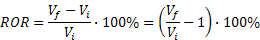where Vi is the initial investment value and Vf is the final investment value.

Annual and Annualized Rate of Return

An Annual Rate of Return is the return on an investment over a one-year period, such as from January 1, 2006 through December 31, 2006. An Annualized Rate of Return is the return on an investment calculated from the return over a period other than one year (such as a month, or two years), using one of the methods described below, to give a comparable one-year return.

### Compound Rate of Return

In financial economics, the term compound ROR indicates a rate of return that is based on compounding, reinvestment, or the changing market value of an account. Compound ROR indicates that the value of the investment increases or decreases during the investment period. To calculate the compound rate of return, the investor includes the reinvested profits in the total investment. The return depends on the frequency of compounding/reinvesting. The table below shows the difference in annual return without/with monthly profit reinvesting. Over a one year period, the difference is small if the annual return is <20%. However, over a multi year period, compounding can make a big difference - please try this calculator.

Annual Rate of Return
Without monthly profit reinvesting (i.e. without monthly change of the nominal account size for notionally funded accounts) With monthly profit reinvesting (the nominal account size is changed monthly to reflect the accumulated profit/loss )
1% 1.005%
5% 5.116%
10% 10.471%
15% 16.075%
20% 21.939%
30% 34.489%
40% 48.213%
50% 63.209%

Calculating Annualized Rates of Return

There are two common ways returns are calculated over multiple periods of time:
• Average Return, or Arithmetic Annualized ROR, or ROR on fixed capital (which can be used if the profit is NOT reinvested), and
• Compound Return or Geometric Annualized ROR (should be used if profit is reinvested); also known as Compound Annual Growth Rate (CAGR). The compound monthly ROR is required by CFTC Regulation 4.35(a)(6)(i)(F) for reporting purposes.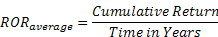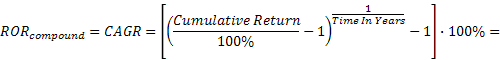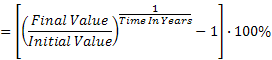Value Added Monthly Index (VAMI) - Hypothetical Growth of \$1,000

The Value Added Monthly Index (VAMI) is an index that tracks the monthly performance of a hypothetical \$1,000 investment using monthly compounding (profit reinvestment) method. The calculation for the n-th month's VAMI is: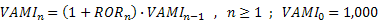where RORn is the monthly ROR for the n-th month and VAMIn is the VAMI index at the end of the n-th month. VAMI is initialized at 1,000.